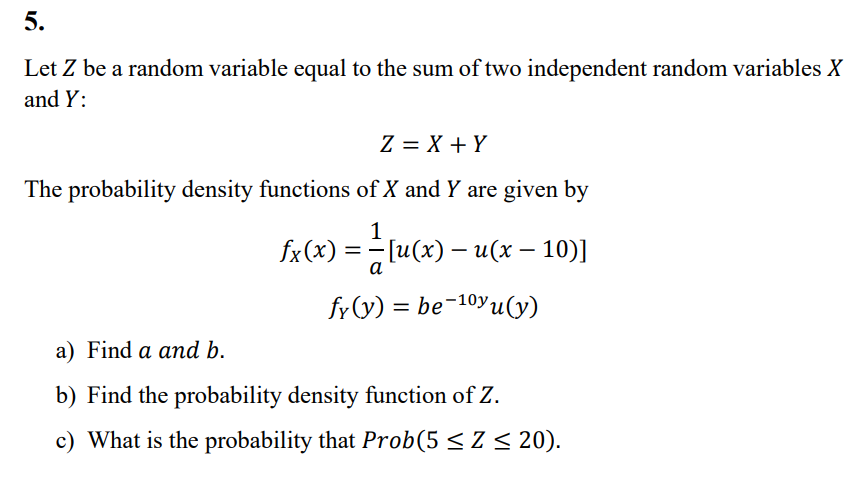Home / Expert Answers / Statistics and Probability / let-z-be-a-random-variable-equal-to-the-sum-of-two-independent-random-variables-x-and-y-z-x-y-th-pa837

# (Solved): Let Z be a random variable equal to the sum of two independent random variables X and Y : Z=X+Y Th ...Let be a random variable equal to the sum of two independent random variables and : The probability density functions of and are given by a) Find and . b) Find the probability density function of . c) What is the probability that .

We have an Answer from Expert

a) To find the values of a and b,
we need to ensure that the probability density functions (PDFs) of X and Y integrate to 1 over their respective support intervals.

Given:

To find a, we integrate   over its support interval   :

so   when   , and 0 otherwise, we have:

To find b, we integrate    over its support interval   :

Since u(y) = 1 when y ? 0, and 0 otherwise, we have:

Applying integration, we get:

b = 10

a is 1/10 and b value is 10.

We have an Answer from Expert